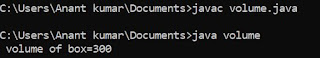## Monday, 2 July 2018

### C Array Types

In C programming array can be divided into two types :

1. Single dimensional array / Simple array / 1-D array / Linear array

2. Multidimensional array

### # Single dimensional array :

When dimension is 1. or when there is a pair of square brackets with the name of array it is known as single dimension array .

Declaration of single dimension array :

data type , variable name ;

### # multidimensional array :

When there are number of pairs of square bracket . or , When there are more than one dimension it is known as multidimensional array . In C programming generally 2- D array are used for the implementation of matrices which is in forms rows and column . In double dimension array first dimension represent the no of rows and second dimension represent the no of columns .

### # Declaration of 2-D array :

Syntax :    data type array name [ no of rows ] [ no of columns ] ;
Or
data type  array name [ m ] [ n ] ;
Where  m = no of rows
n = no of columns
Example :

int a   ;
1 element = a  
2 element = a  
3 element = a  
4 element = a  
5 element = a [1[ 
6 element = a  
7 element = a  
8 element = a  
9 element = a  

### # Input and output with double dimensional array :

# include <stdio.h>
void main ()
{
int a   ;
for ( i = 0 ; i < 3 ; i++ )
{
for ( j = 0 ; j < 3 ; j++ )
{
printf ("\n Enter a number:") ;
scanf ("%d", & a[i] [j]) ;
}

### #  Output with 2 - D array :

for ( i = 0 ; i < n ; i++ )
{
for ( j = 0 ; j < n ; j++ )
{
printf ("%d", a [i] [j]) ;
printf ("\n") ;
}
}